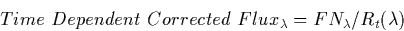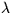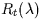The degradation ratio at 1985.0, which corresponds to the mean time of the ITFs, was calculated for each wavelength bin using the linear fits. A zeropoint correction was then applied to the y-intercepts in order to force the degradation ratios to be one. This ensures that no degradation correction will be applied to data near the 1985.0 fiducial date.

The ratios are applied to the net flux spectra using a nearest neighbor wavelength interpolation scheme along with the inverse sensitivity function so as to provide absolutely calibrated and degradation-corrected flux data. Extended source images are corrected using the point source ratios. The correction is performed in the following manner:

• The appropriate coefficients which correspond to the calibration epoch of the observation in question are identified. In the case of the LWR camera, there is only one calibration epoch.
• A correction ratio for each wavelength is calculated in the following manner:

Rt = R0 + R1 D + R2 D2 + R3 D3 + R4 D4

where D is the observation date expressed as either decimal years (e.g., 1984.3) for the LWP and SWP or decimal years minus 1978.0 (e.g., 6.3 for an observation date of 1984.3) for the LWR. In addition, the coefficients R2, R3, and R4 are zero for the LWP and SWP.
• The correction ratio is applied as follows:whererefers to the closest 5Å bin andis the Rt value for that bin.